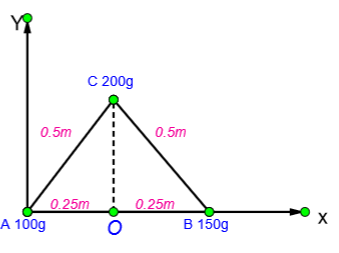QuestionAnswers

# Find centre of mass of three particles at the vertices of an equilateral triangle. The masses of the particle are 100g, 150g, and 200g respectively. Each side of the equilateral triangle is 0.5 m long.

Hint: We have to find the position centre of mass of three particles. To find this, we first find the coordinates of the position of the given three masses. And then we substitute the values in the known equation used to find the position of centre of mass.

Formula used: The position of centre of mass is given by the equation,
${{x}_{cm}}=\dfrac{{{m}_{1}}{{x}_{1}}+{{m}_{2}}{{x}_{2}}+{{m}_{3}}{{x}_{3}}}{{{m}_{1}}+{{m}_{2}}+{{m}_{3}}}$ And ${{y}_{cm}}=\dfrac{{{m}_{1}}{{y}_{1}}+{{m}_{2}}{{y}_{2}}+{{m}_{3}}{{y}_{3}}}{{{m}_{1}}+{{m}_{2}}+{{m}_{3}}}$

In the question we are given three particles which are placed at the vertices of an equilateral triangle.
Mass of all three particles is given,
\begin{align} & {{m}_{1}}=100g \\ & {{m}_{2}}=150g \\ & {{m}_{3}}=200g \\ \end{align}
Length of each side of the triangle is 0.5 m.
The three masses are placed on an equilateral triangle as shown below.ABC is the equilateral triangle. OC is the perpendicular drawn from C to AB. Hence OC divides the side AB in half.
Therefore$AO=OB=\dfrac{0.5}{2}=0.25m$, and we know that $AC=CB=0.5$ .
Now, from the figure we can find the coordinates of the points A, B and C.
A is at the origin, hence the coordinate of A is $\left( 0,0 \right)$.
B is on the X-axis; hence the y- coordinate of B is 0. X coordinate of B is the length of AB, i.e. 0.5 m (given in the question)
Therefore the coordinate of B is $\left( 0.5,0 \right)$.
To find the coordinates of C, let us consider the right angled triangle AOC.
We know that,
\begin{align} & AC=0.5 \\ & AO=0.25 \\ \end{align}
According to Pythagoras theorem, the square of hypotenuse is the sum of the square of the other two sides.
$hypotnus{{e}^{2}}=bas{{e}^{2}}+heigh{{t}^{2}}$
Here, we can write this as,
$A{{C}^{2}}=A{{O}^{2}}+O{{C}^{2}}$
Here we know AC and AO. We have to find OC. For that, we can rewrite the equation as
$O{{C}^{2}}=A{{C}^{2}}-A{{O}^{2}}$
By applying the values of AC and AO in this equation, we get
$O{{C}^{2}}=({{0.5}^{2}})-({{0.25}^{2}})$
$O{{C}^{2}}=0.25-0.0625=0.1875$
$OC=\sqrt{0.1875}$
$OC=0.25\sqrt{3}$
This is the y coordinate of C. X coordinate of C is the length of $AO=0.25$.
Therefore coordinates of C is $\left( 0.25,0.25\sqrt{3} \right)$.
Now that we have all three coordinates, we have to find the position coordinates of the centre of mass of these particles.
To find the centre of mass of 3 particles, we have the equation
To find the x coordinate,
${{x}_{cm}}=\dfrac{{{m}_{1}}{{x}_{1}}+{{m}_{2}}{{x}_{2}}+{{m}_{3}}{{x}_{3}}}{{{m}_{1}}+{{m}_{2}}+{{m}_{3}}}$
We have, ${{m}_{1}}=100,{{m}_{2}}=150,{{m}_{3}}=200$ and ${{x}_{1}}=0,{{x}_{2}}=0.5,{{x}_{3}}=0.25$
Therefore,
${{x}_{cm}}=\left( \dfrac{\left( 100\times 0 \right)+\left( 150\times 0.5 \right)+\left( 200\times 0.25 \right)}{100+150+200} \right)$
${{x}_{cm}}=\left( \dfrac{0+75+50}{450} \right)$
By solving this, we get
${{x}_{cm}}=0.27778\approx 0.28m$
To find y coordinate, the equation is
${{y}_{cm}}=\dfrac{{{m}_{1}}{{y}_{1}}+{{m}_{2}}{{y}_{2}}+{{m}_{3}}{{y}_{3}}}{{{m}_{1}}+{{m}_{2}}+{{m}_{3}}}$
Here, ${{y}_{1}}=0,{{y}_{2}}=0,{{y}_{3}}=0.25\sqrt{3}$
Therefore,
${{y}_{cm}}=\left( \dfrac{\left( 100\times 0 \right)+\left( 150\times 0 \right)+\left( 200\times 0.25\sqrt{3} \right)}{450} \right)$
${{y}_{cm}}=\dfrac{50\sqrt{3}}{450}$
By solving this we get,
${{y}_{cm}}=0.192\approx 0.2m$
Therefore, the centre of mass of the three particles at the vertices of an equilateral triangle is at,\begin{align} & x=0.28m \\ & y=0.2m \\ \end{align}

Note: The centre of mass of a system of particles or a body is the point at which the whole mass of the body or the system is concentrated. The whole weight of the object is concentrated at this point. The position of centre of mass varies for every body.
Equilateral triangle is a triangle with all the three sides equal. An equilateral triangle is also equiangular, i.e. for an equilateral triangle all three internal angles are equal to ${{60}^{o}}$. Since all three sides and all three angles are equal, the perpendicular drawn to one side bisects that side.